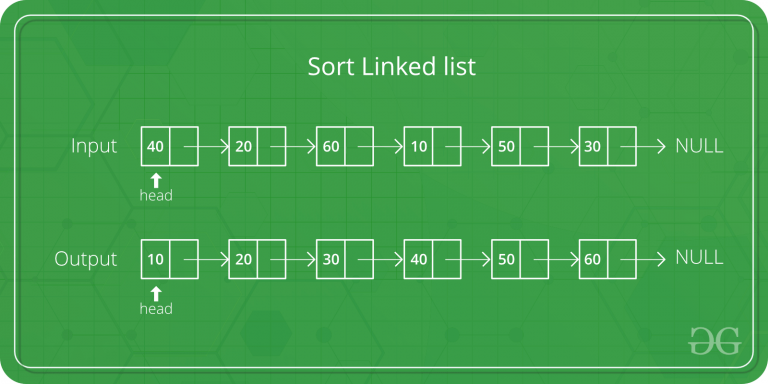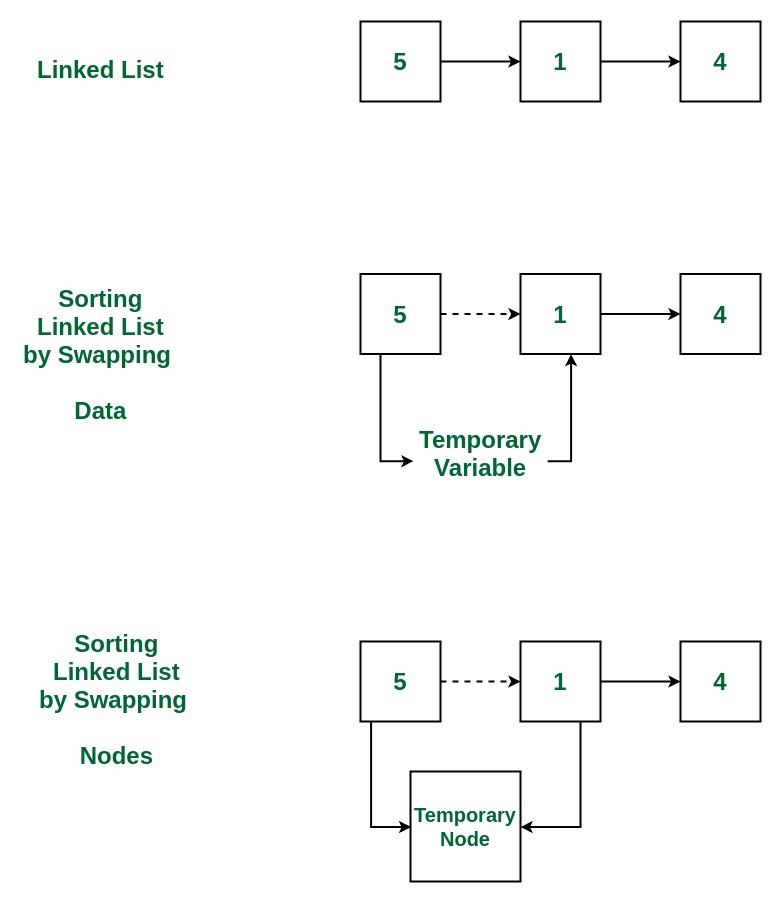# Bubble Sort for Linked List by Swapping nodes

Given a singly linked list, sort it using bubble sort by swapping nodes.Example:

```Input: 10->30->20->5
Output: 5->10->20->30

Input: 20->4->3
Output: 3->4->20```## Recommended: Please try your approach on {IDE} first, before moving on to the solution.

Approach:

1. Get the Linked List to be sorted
2. Apply Bubble Sort to this linked list, in which, while comparing the two adjacent nodes, actual nodes are swapped instead of just swapping the data.
3. Print the sorted list

Below is the implementation of the above approach:

## C++

 `// C++ program to sort Linked List ` `// using Bubble Sort ` `// by swapping nodes ` ` `  `#include ` `using` `namespace` `std; ` ` `  `/* structure for a node */` `struct` `Node  ` `{ ` `    ``int` `data; ` `    ``struct` `Node* next; ` `} Node; ` ` `  `/*Function to swap the nodes */` `struct` `Node* swap(``struct` `Node* ptr1, ``struct` `Node* ptr2) ` `{ ` `    ``struct` `Node* tmp = ptr2->next; ` `    ``ptr2->next = ptr1; ` `    ``ptr1->next = tmp; ` `    ``return` `ptr2; ` `} ` ` `  `/* Function to sort the list */` `int` `bubbleSort(``struct` `Node** head, ``int` `count) ` `{ ` `    ``struct` `Node** h; ` `    ``int` `i, j, swapped; ` ` `  `    ``for` `(i = 0; i <= count; i++) ` `    ``{ ` ` `  `        ``h = head; ` `        ``swapped = 0; ` ` `  `        ``for` `(j = 0; j < count - i - 1; j++)  ` `        ``{ ` ` `  `            ``struct` `Node* p1 = *h; ` `            ``struct` `Node* p2 = p1->next; ` ` `  `            ``if` `(p1->data > p2->data) ` `            ``{ ` ` `  `                ``/* update the link after swapping */` `                ``*h = swap(p1, p2); ` `                ``swapped = 1; ` `            ``} ` ` `  `            ``h = &(*h)->next; ` `        ``} ` ` `  `        ``/* break if the loop ended without any swap */` `        ``if` `(swapped == 0) ` `            ``break``; ` `    ``} ` `} ` ` `  `/* Function to print the list */` `void` `printList(``struct` `Node* n) ` `{ ` `    ``while` `(n != NULL) ` `    ``{ ` `        ``cout << n->data << ``" -> "``; ` `        ``n = n->next; ` `    ``} ` `    ``cout << endl; ` `} ` ` `  `/* Function to insert a struct Node ` `at the beginning of a linked list */` `void` `insertAtTheBegin(``struct` `Node** start_ref, ``int` `data) ` `{ ` `    ``struct` `Node* ptr1 ` `        ``= (``struct` `Node*)``malloc``(``sizeof``(``struct` `Node)); ` ` `  `    ``ptr1->data = data; ` `    ``ptr1->next = *start_ref; ` `    ``*start_ref = ptr1; ` `} ` ` `  `// Driver Code ` `int` `main() ` `{ ` `    ``int` `arr[] = { 78, 20, 10, 32, 1, 5 }; ` `    ``int` `list_size, i; ` ` `  `    ``/* start with empty linked list */` `    ``struct` `Node* start = NULL; ` `    ``list_size = ``sizeof``(arr) / ``sizeof``(arr); ` ` `  `    ``/* Create linked list from the array arr[] */` `    ``for` `(i = 0; i < list_size; i++) ` `        ``insertAtTheBegin(&start, arr[i]); ` ` `  `    ``/* print list before sorting */` `    ``cout <<``"Linked list before sorting\n"``; ` `    ``printList(start); ` ` `  `    ``/* sort the linked list */` `    ``bubbleSort(&start, list_size); ` ` `  `    ``/* print list after sorting */` `    ``cout <<``"Linked list after sorting\n"``; ` `    ``printList(start); ` ` `  `    ``return` `0; ` `} ` ` `  `// This code is contributed by ` `// shubhamsingh10 `

## C

 `// C program to sort Linked List ` `// using Bubble Sort ` `// by swapping nodes ` ` `  `#include ` `#include ` ` `  `/* structure for a node */` `struct` `Node { ` `    ``int` `data; ` `    ``struct` `Node* next; ` `} Node; ` ` `  `/*Function to swap the nodes */` `struct` `Node* swap(``struct` `Node* ptr1, ``struct` `Node* ptr2) ` `{ ` `    ``struct` `Node* tmp = ptr2->next; ` `    ``ptr2->next = ptr1; ` `    ``ptr1->next = tmp; ` `    ``return` `ptr2; ` `} ` ` `  `/* Function to sort the list */` `int` `bubbleSort(``struct` `Node** head, ``int` `count) ` `{ ` `    ``struct` `Node** h; ` `    ``int` `i, j, swapped; ` ` `  `    ``for` `(i = 0; i <= count; i++) { ` ` `  `        ``h = head; ` `        ``swapped = 0; ` ` `  `        ``for` `(j = 0; j < count - i - 1; j++) { ` ` `  `            ``struct` `Node* p1 = *h; ` `            ``struct` `Node* p2 = p1->next; ` ` `  `            ``if` `(p1->data > p2->data) { ` ` `  `                ``/* update the link after swapping */` `                ``*h = swap(p1, p2); ` `                ``swapped = 1; ` `            ``} ` ` `  `            ``h = &(*h)->next; ` `        ``} ` ` `  `        ``/* break if the loop ended without any swap */` `        ``if` `(swapped == 0) ` `            ``break``; ` `    ``} ` `} ` ` `  `/* Function to print the list */` `void` `printList(``struct` `Node* n) ` `{ ` `    ``while` `(n != NULL) { ` `        ``printf``(``"%d -> "``, n->data); ` `        ``n = n->next; ` `    ``} ` `    ``printf``(``"\n"``); ` `} ` ` `  `/* Function to insert a struct Node ` `   ``at the beginning of a linked list */` `void` `insertAtTheBegin(``struct` `Node** start_ref, ``int` `data) ` `{ ` `    ``struct` `Node* ptr1 ` `        ``= (``struct` `Node*)``malloc``(``sizeof``(``struct` `Node)); ` ` `  `    ``ptr1->data = data; ` `    ``ptr1->next = *start_ref; ` `    ``*start_ref = ptr1; ` `} ` ` `  `// Driver Code ` `int` `main() ` `{ ` `    ``int` `arr[] = { 78, 20, 10, 32, 1, 5 }; ` `    ``int` `list_size, i; ` ` `  `    ``/* start with empty linked list */` `    ``struct` `Node* start = NULL; ` `    ``list_size = ``sizeof``(arr) / ``sizeof``(arr); ` ` `  `    ``/* Create linked list from the array arr[] */` `    ``for` `(i = 0; i < list_size; i++) ` `        ``insertAtTheBegin(&start, arr[i]); ` ` `  `    ``/* print list before sorting */` `    ``printf``(``"Linked list before sorting\n"``); ` `    ``printList(start); ` ` `  `    ``/* sort the linked list */` `    ``bubbleSort(&start, list_size); ` ` `  `    ``/* print list after sorting */` `    ``printf``(``"Linked list after sorting\n"``); ` `    ``printList(start); ` ` `  `    ``return` `0; ` `} `

Output:

```Linked list before sorting
5 -> 1 -> 32 -> 10 -> 20 -> 78 ->
1 -> 5 -> 10 -> 20 -> 32 -> 78 ->
```

Don’t stop now and take your learning to the next level. Learn all the important concepts of Data Structures and Algorithms with the help of the most trusted course: DSA Self Paced. Become industry ready at a student-friendly price.

My Personal Notes arrow_drop_upCheck out this Author's contributed articles.

If you like GeeksforGeeks and would like to contribute, you can also write an article using contribute.geeksforgeeks.org or mail your article to contribute@geeksforgeeks.org. See your article appearing on the GeeksforGeeks main page and help other Geeks.

Please Improve this article if you find anything incorrect by clicking on the "Improve Article" button below.

Improved By : SHUBHAMSINGH10

Article Tags :
Practice Tags :

3

Please write to us at contribute@geeksforgeeks.org to report any issue with the above content.